# PSAT Math : How to find the length of the hypotenuse of a right triangle : Pythagorean Theorem

## Example Questions

1 2 3 4 6 Next →

### Example Question #51 : How To Find The Length Of The Hypotenuse Of A Right Triangle : Pythagorean Theorem

Susie walks north from her house to a park that is 30 meters away. Once she arrives at the park, she turns and walks west for 80 meters to a bench to feed some pigeons. She then walks north for another 30 meters to a concession stand. If Susie returns home in a straight line from the concession stand, how far will she walk from the concession stand to her house, in meters?

200

70

100

50

25

100

Explanation:

Susie walks 30 meters north, then 80 meters west, then 30 meters north again. Thus, she walks 60 meters north and 80 meters west. These two directions are 90 degrees away from one another.

At this point, construct a right triangle with one leg that measures 60 meters and a second leg that is 80 meters.

You can save time by using the 3:4:5 common triangle. 60 and 80 areand, respectively, making the hypotenuse equal to.

We can solve for the length of the missing hypotenuse by applying the Pythagorean theorem: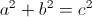Substitute the following known values into the formula and solve for the missing hypotenuse: side.Susie will walk 100 meters to reach her house.

### Example Question #161 : Triangles

The lengths of the sides of a triangle are consecutive odd numbers and the triangle's perimeter is 57 centimeters. What is the length, in centimeters, of its longest side?

25

19

23

17

21

21

Explanation:

First, define the sides of the triangle. Because the side lengths are consecutive odd numbers, if we define the shortest side will be as, the next side will be defined as, and the longest side will be defined as. We can then find the perimeter of a triangle using the following formula:Substitute in the known values and variables.Subtract 6 from both sides of the equation.Divide both sides of the equation by 3.Solve.This is not the answer; we need to find the length of the longest side, orSubstitute in the calculated value forand solve.The longest side of the triangle is 21 centimeters long.

### Example Question #21 : Right Triangles

Each of the following answer choices lists the side lengths of a different triangle.  Which of these triangles does not have a right angle?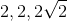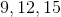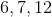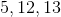cannot be the side lengths of a right triangle.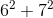does not equal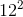. Also, special right triangle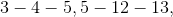and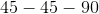rules can eliminate all the other choices.